﻿ 非线性半定规划一个新的全局收敛算法

# 非线性半定规划一个新的全局收敛算法A New Globally Convergent Algorithm for Nonlinear Semidefinite Programming

Abstract: In this paper, we present a sequence quadratic semidefinite programming (SSDP) algorithm for nonlinear semidefinite programming. At each iteration, the search direction is determined by solving two semidefinite programming sub problems; by introducing a distance function, a merit function is constructed for line search. Under some appropriate conditions, any accumulation point of iterative point sequence is either an infeasible stationary point, or a KKT point of the problem.

1. 引言

$\begin{array}{l}\mathrm{min}\begin{array}{cc}& f\left(x\right)\end{array}\\ \text{s}\text{.t}\text{.}\text{ }\text{ }\mathcal{A}\left(x\right)\underset{_}{\prec }0,\\ \begin{array}{ccc}& & \text{ }\text{\hspace{0.17em}}h\left(x\right)\end{array}=0,\end{array}$ (1)

· 产生搜索方向的子问题是相容的；

· 初始点任意，并且算法的线搜索保证效益函数的充分下降；

· 在一定条件下算法或收敛到问题的不可行稳定点，或收敛到问题的KKT点。

2. 算法

${ℝ}^{m×n}$ 为所有 $m×n$ 矩阵构成的集合， ${\mathbb{S}}_{+}^{m}\left({\mathbb{S}}_{++}^{m}\right)$ 为所有对称半正定(正定)矩阵构成的集合， ${\mathbb{S}}_{-}^{m}\left({\mathbb{S}}_{--}^{m}\right)$ 为所有对称半负定(负定)矩阵构成的集合。记NLSDP (0.1)的可行集为S。

$〈A,B〉:=\text{Tr}\left({B}^{\text{T}}A\right)=\underset{i=1}{\overset{m}{\sum }}\underset{j=1}{\overset{n}{\sum }}{a}_{ij}{b}_{ij},$

$Dh\left(x\right)=\left(\begin{array}{ccc}\frac{\partial {h}_{1}\left(x\right)}{\partial {x}_{1}}& \cdots & \frac{\partial {h}_{1}\left(x\right)}{\partial {x}_{n}}\\ ⋮& \ddots & ⋮\\ \frac{\partial {h}_{{m}_{2}}\left(x\right)}{\partial {x}_{1}}& \cdots & \frac{\partial {h}_{{m}_{2}}\left(x\right)}{\partial {x}_{n}}\end{array}\right).$ (1.1)

$D\mathcal{A}\left(x\right)={\left(\frac{\partial \mathcal{A}\left(x\right)}{\partial {x}_{1}},\cdots ,\frac{\partial \mathcal{A}\left(x\right)}{\partial {x}_{n}}\right)}^{\text{T}},$

$D\mathcal{A}\left(x\right)d:=\underset{i=1}{\overset{n}{\sum }}{d}_{i}\frac{\partial \mathcal{A}\left(x\right)}{\partial {x}_{i}}.$ (1.2)

${V}^{*}Z={\left(〈{V}_{1},Z〉,\cdots ,〈{V}_{n},Z〉\right)}^{\text{T}},\forall Z\in {\mathbb{S}}^{{m}_{1}}.$

NLSDP (0.1)的Lagrange函数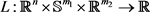定义为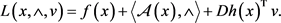(1.3)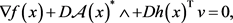(1.4a)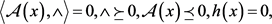(1.4b)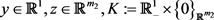，定义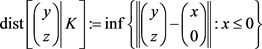到集合K的距离，其中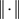表示向量的欧式范数。(1.5)(1.6)(1.7)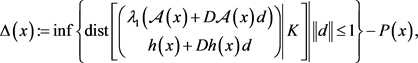(1.8)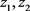不全为0，且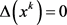，则算法终止，否则转步骤2；(1.9)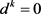，则算法停止；否则，转步骤3。(1.10)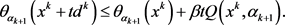(1.11)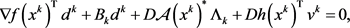(1.2a)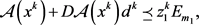(1.12b)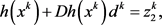(1.12c)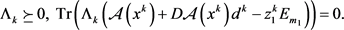(1.12d)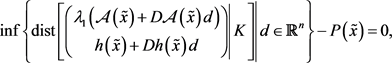A1：函数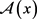是连续可微的。

A2：存在正数a和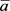使得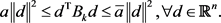A3：由算法A产生的迭代点列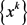是有界的。

A4：对任意的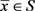，Mangasarian-Fromovitz约束规格(MFCQ)成立，即

1)行满秩；

2) 存在非零向量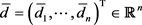，使得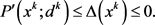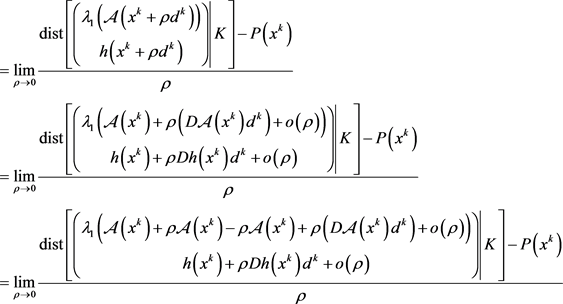，此时若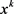为NLSDP (0.1)的不可行点，由不可行稳定点的定义知为NLSDP (0.1)的不可行稳定点。因此综合以上所述引理结论得证。,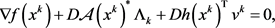(1.13a)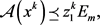(1.13b)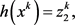(1.13c)(1.13d),

3. 全局收敛性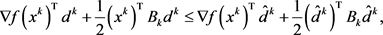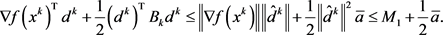(2.1)(2.2)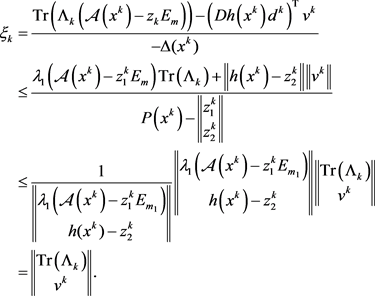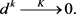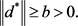(2.3)

a) 证明存在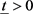，使得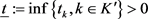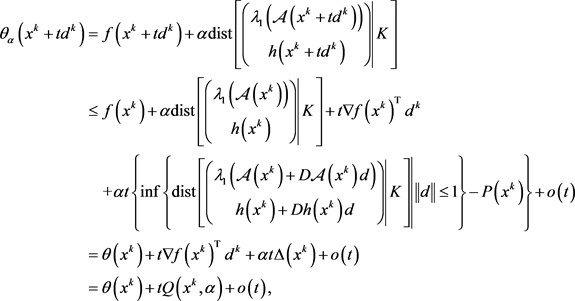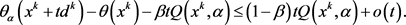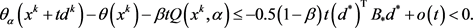(2.4)

b) 基于，将导出矛盾。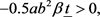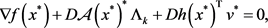(2.5a)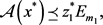(2.5b)(2.5c)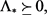(2.5d)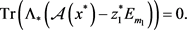(2.5e)

4. 结束语

NOTES

*通讯作者。

 Ben-Tal, A., Jarre, F., Kocvara, M., Nemirovski, A. and Zowe, J. (2000) Optimal Design of Trusses under a Nonconvex Global Buckling Constraint. Optimization and Engineering, 1, 189-213.
https://doi.org/10.1023/A:1010091831812

 Fares, B., Noll, D. and Apkarian, P. (2002) Robust Control via Sequential Semidefinite Programming. SIAM Journal on Control and Optimization, 40, 1791-1820.
https://doi.org/10.1137/S0363012900373483

 Kocvara, M. and Stingl, M. (2004) Solving Nonconvex SDP Problems of Structural Optimization with Stability Control. Optimization Methods and Software, 19, 595-609.
https://doi.org/10.1080/10556780410001682844

 Jarre, F. (2000) An Interior Method for Nonconvex Semidefinite Programs. Optimization and Engineering, 1, 347-372.
https://doi.org/10.1023/A:1011562523132

 Fares, B., Apkarian, P. and Noll, D. (2001) An Augmented Lagrangian Method for a Class of LMI-Constrained Problems in Robust Control Theory. International Journal of Control, 74, 3702-3706.
https://doi.org/10.1080/00207170010010605

 Nishimura, R., Hayashi, S. and Fukushima, M. (2012) Semidefinite Complement Arit Reformulation for Robust Nash Equilibrium Problems with Euclidean Uncertainty Sets. Journal of Global Optimization, 53, 107-120.

 Correa, R. and Ramirez, H. (2004) A Global Algorithm for Nonlinear Semidefinite Programming. SIAM Journal on Optimization, 15, 303-318.
https://doi.org/10.1137/S1052623402417298

 Burke, J.V. and More, J.J. (1988) On the Identification of Active Constraints. SIAM Journal on Numerical Analysis, 25, 1197-1211.
https://doi.org/10.1137/0725068

 Dunn, J.C. (1987) On the Convergence of Projected Gradient Processes to Singular Critical Points. Journal of Optimization Theory and Applications, 55, 203-216.
https://doi.org/10.1007/BF00939081

 Burke, J.V. and Han, S.P. (1989) A Robust Sequential Quadratic Programming Method. Mathematical Programming, 43, 277-303.
https://doi.org/10.1007/BF01582294

Top